# Intuitionistic fuzzy sets

(diff) ← Older revision | Latest revision (diff) | Newer revision → (diff)

Let us have a fixed universe$E$ and its subset$A$. The set$A^* = \lbrace \langle x, \mu_A(x), \nu_A(x) \rangle \ | \ x \in E \rbrace$

where$0 \leq \mu_A(x) + \nu_A(x) \leq 1$ is called intuitionistic fuzzy set.

Functions$\mu_A: E \to [0,1]$ and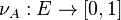$\nu_A: E \to [0,1]$ represent degree of membership (validity, etc.) and non-membership (non-validity, etc.).

We can define also function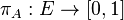$\pi_A: E \to [0,1]$ through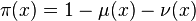$\pi(x) = 1 - \mu (x) - \nu (x)$

and it corresponds to degree of indeterminacy (uncertainty, etc.).

For brevity, we shall write below$A$ instead of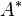$A^*$, whenever this is possible.

Obviously, for every ordinary fuzzy set$A$:$\pi_A(x) = 0$ for each$x \in E$ and these sets have the form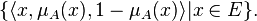$\lbrace \langle x, \mu_{A}(x), 1-\mu_{A}(x)\rangle |x \in E \rbrace.$